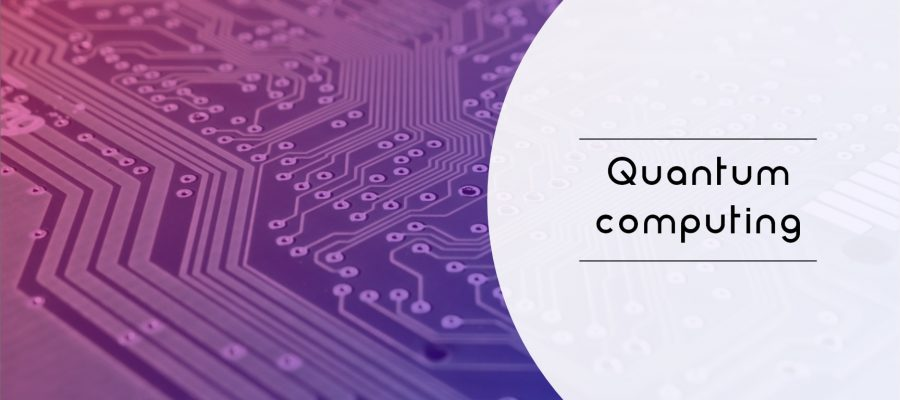# Introduction to Quantum Computing

Quantum computing is the use of quantum-mechanical phenomena such as superposition and entanglement to perform computation. A quantum computer is used to perform such computation, which can be implemented theoretically or physically.

The field of quantum computing is actually a sub-field of quantum information science, which includes quantum cryptography and quantum communication. Quantum Computing was started in the early 1980s when Richard Feynman and Yuri Manin expressed the idea that a quantum computer had the potential to simulate things that a classical computer could not. In 1994, Peter Shor shocked the world with an algorithm that had the potential to decrypt all secured communications.

There are two main approaches to physically implementing a quantum computer currently, analog and digital. Analog approaches are further divided into quantum simulation, quantum annealing, and adiabatic quantum computation. Digital quantum computers use quantum logic gates to do computation. Both approaches use quantum bits or qubits.

Qubits are fundamental to quantum computing and are somewhat analogous to bits in a classical computer. Qubits can be in a 1 or 0 quantum state. But they can also be in a superposition of the 1 and 0 states. However, when qubits are measured the result is always either a 0 or a 1; the probabilities of the two outcomes depends on the quantum state they were in.

Today’s physical quantum computers are very noisy and quantum error correction is a burgeoning field of research. Unfortunately existing hardware is so noisy that fault-tolerant quantum computing [is] still a rather distant dream. As of April 2019 neither large scalable quantum hardware has been demonstrated nor have commercially useful algorithms for today’s small noisy quantum computers been published.There is an increasing amount of investment in quantum computing by governments, established companies, and start-ups. Both applications of near-term intermediate-scale device and the demonstration of quantum supremacy are actively pursued in academic and industrial research.

A classical computer has a memory made up of bits, where each bit is represented by either a one or a zero. A quantum computer, on the other hand, maintains a sequence of qubits, which can represent a one, a zero, or any quantum superposition of those two qubit states; a pair of qubits can be in any quantum superposition of 4 states, and three qubits in any superposition of 8 states. In general, a quantum computer with {\displaystyle n}qubits can be in any superposition of up to {\displaystyle 2^{n}}different states. (This compares to a normal computer that can only be in one of these {\displaystyle 2^{n}}states at any one time).

A quantum computer operates on its qubits using quantum gates and measurement (which also alters the observed state). An algorithm is composed of a fixed sequence of quantum logic gates and a problem is encoded by setting the initial values of the qubits, similar to how a classical computer works. The calculation usually ends with a measurement, collapsing the system of qubits into one of the {\displaystyle 2^{n}}eigenstates, where each qubit is zero or one, decomposing into a classical state. The outcome can, therefore, be at most {\displaystyle n}classical bits of information. If the algorithm did not end with a measurement, the result is an unobserved quantum state. (Such unobserved states may be sent to other computers as part of distributed quantum algorithms.)

Quantum algorithms are often probabilistic, in that they provide the correct solution only with a certain known probability. Note that the term non-deterministic computing must not be used in that case to mean probabilistic (computing) because the term non-deterministic has a different meaning in computer science.

An example of an implementation of qubits of a quantum computer could start with the use of particles with two spin states: “down” and “up” (typically written {\displaystyle |{\downarrow }\rangle }and {\displaystyle |{\uparrow }\rangle }, or {\displaystyle |0{\rangle }}and {\displaystyle |1{\rangle }}). This is true because any such system can be mapped onto an effective spin-1/2 system.

The above is the basic of Quantum Computing. Watch this space for more updates on the latest trends in Technology.